# KSEEB Class 8 Science Important Questions Chapter 15 Heat

Students can download Class 8 Science Chapter 15 Heat Important Questions, KSEEB Class 8 Science Important Questions and Answers helps you to revise the complete Karnataka State Board Syllabus and to clear all their doubts, score well in final exams.

## Karnataka State Syllabus Class 8 Science Important Questions Chapter 15 Heat

Question 1.
What is heat? Mention some of the sources of heat.
Heat is a form of energy. It is the sum of the kinetic energies of all the molecules of a substance. The Sun is the biggest natural source of heat to us. Other than the sun, burning of fuels, friction, electricity etc., are also some of the sources of heat.Question 2.
What is the S.I. unit of heat?
The S.I. unit of heat is called ‘joule’ (J).

Question 3.
What is temperature? What does it depend on?
The degree of hotness or coldness of an object is called its temperature. The temperature of a substance depends on the average kinetic energy of its molecules.

Question 4.
What happens to the kinetic energy of the molecules of a substance when heat is added to or removed from it?
When heat is added to a substance, the kinetic energy of its molecules will increase. When heat is removed from a substance, the random kinetic energy of its molecules will decrease.

Question 5.
Give one example to illustrate that heat can be generated from the energy of motion.
When we rub our palms vigorously, heat is produced. Here, mechanical energy (energy of motion) is changed into heat energy.

Question 6.
Mention the various units of temperature commonly used. Which is the SJ. unit of temperature?
The commonly used units of temperature are ‘degree Fahrenheit’ (°F) and ‘degree Celsius’ (°C). The S.I. unit of temperature is ‘kelvin’ (K).

Question 7.
Distinguish between heat and temperature.
Heat:

1. Heat is a form of energy.
2. Heat energy depends upon the speed of the particles, the number of particles and the mass of an object.
3. The S.I. unit of heat is called ‘joule’.

Temperature:

1. Temperature is the degree of hotness or coldness of an object.
2. Temperature does not depend on the mass of an object.
3. The S.I. unit of temperature is called ‘kelvin’.

Question 8.
List the three different effects produced by heat.
The various effects of heat are

• Thermal expansion
• Increase in temperature
• Change of physical state.

Question 9.
What is meant by thermal expansion?
Generally all substances expand on heating and contract on cooling. The increase in the dimensions of an object due to heating is called thermal expansion.Question 10.
Describe an experiment to show the change in state on heating.
Take two aluminium rods about 7 cm each. Connect the two rods between two supports as shown in the figure. Leave a paper-thin gap between the two rods.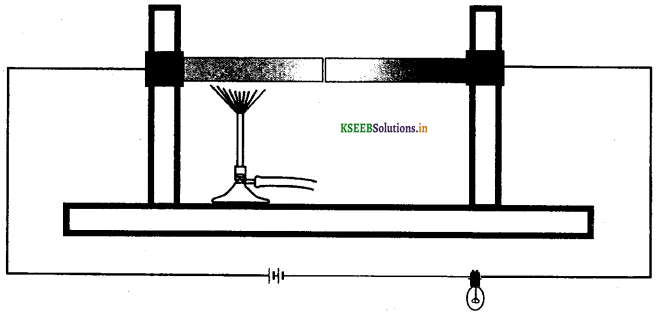Connect the two rods to a bulb and a battery. The bulb does not glow now as the circuit is not completed because of the gap between the rods. Now heat the aluminium rods with a spirit lamp. Now the bulb begins to glow. On cooling the rods, the bulb again stops glowing. This shows that the aluminium rod has expanded on heating.

Question 11.
What is a bi-metallic strip? Name any two devices which use bimetallic strip.
Two strips made of different metals and bound together with rivets is called a bi-metallic strip. Bi-metallic strips are used in fire alarms, thermal switches, electric iron, electric oven, electric heater, geyser, etc.

Question 12.
A thick glass tumbler often cracks when boiling water is poured into it. Why?
When boiling water is poured into a thick glass tumbler, the inner surface of the glass becomes hot and expands. However, the outer surface of the tumbler does not expand much as glass is a bad conductor of heal. This uneven expansion of glass causes the tumbler to develop cracks.

Question 13.
Give an example of a glass that is resistant to cracks on heating.
Pyrex glass resists cracks on heating. This is because the expansion due to heating is quite low for Pyrex glass.

Question 14.
Why is a small space left between the concrete blocks while making cement roads?
A small gap is left between concrete blocks while laying roads to accommodate for thermal expansion during summer days. If such a gap is not left, the blocks develop stress and crack.

Question 15.
Why are metal pipelines meant to carry hot liquids provided with loops at regular intervals?
In industries, hot liquids are transported from place to place using metal pipelines. The pipelines may break due to the stress developed by expansion and contraction of the pipes. This problem is overcome by providing loops to the pipelines at regular intervals. The thermal expansion causes the loop to expand only slightly. Hence, the pipe does not break.

Question 16.
Describe a simple activity to show that gases expand on het.
Take a small round bottomed flask fitted with a one holed rubber cork. Fix a long glass tube through the hole. Introduce a drop of coloured water into the glass tube. Hold the flask tightly. Observe the movement of the water drop. The water drop moves up due to the expansion of the air inside the flask due to heating.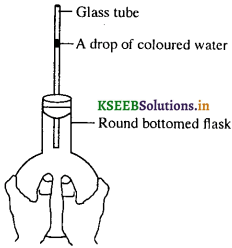Question 17.
What is the principle of working of an automobile engine?
An automobile engine converts heat energy into mechanical work. It works on the principle that gases expand on heating. The expanding hot gases do mechanical work.Question 18.
Give any one practical application of thermal expansion of liquids.
Thermal expansion of liquids is used to make mercury thermometers. Mercury expands on heating and contracts on cooling. This principle is used in construction of mercury thermometers.

Question 19.
What is anomalous expansion of water?
Usually substances expand on heating and contract on cooling. However, water shows an exception to this between 0 °C and 4 °C. As we go on increasing the temperature from 0 °C to 4 °C, water contracts. Water expands as we cool from 4 °C to 0 °C. This unusual behaviour of water between 0 °C and 4 °C is called anomalous expansion of water.

Question 20.
What advantage is provided by anomalous expansion of water to aquatic life in cold weather?
The anomalous expansion of water is useful for the preservation of marine life in extremely cold temperatures. Initially, the surface water in water bodies starts cooling. The heavier water moves to the bottom. Upon further cooling between 0 °C and 4 °C, the bottom-most layer is at 4 °C and the temperature gradually drops as one goes upwards.

At 0 °C, the water at the surface gradually freezes into ice and floats on the surface of water but the water under the ice layer remains at 4 °C. Since ice is a poor conductor of heat, it checks the transfer of heat from the water of the lake below the layer of ice and thus, helps in keeping the water under the layer of ice at 4 °C. Consequently, the fish and other aquatic life survive in water bodies though their surface has frozen into ice.

Question 21.
Why does ice float on water?
The density of ice is slightly lower than that of water. This is because, for the same mass of water ice has higher volume. This is due to anomalous expansion of water. Therefore, ice floats on water.

Question 22.
What is a thermometer?
An instrument used to measure the temperature of a substance is called a thermometer.

Question 23.
What is the type of thermometer generally used in laboratories and in day-to-day life?
Generally, mercury thermometers are used in laboratories and in day-to-day life.

Question 24.
State the similarities between the laboratory thermometer and the clinical thermometer.
Both thermometers use mercury. They work on the same principle that mercury expands on heating.

Question 25.
State two differences between a laboratory mercury thermometer and a clinical thermometer.
A clinical thermometer has a limited range and there is a constriction in the capillary tube just above the bulb. The range of laboratory’ thermometer is much higher than that of a clinical thermometer. Secondly, there is no constriction in the capillary’ tube in the case of a laboratory thermometer.Question 26.
What is the principle of a mercury thermometer?
A mercury thermometer is based on the property of expansion of liquids on heating. Mercury expands on heating and contracts on cooling.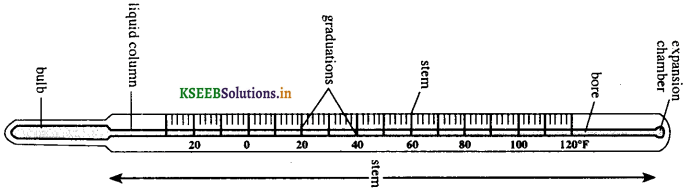Question 27.
With the help of a neat diagram, explain briefly the construction of a laboratory or mercury thermometer.
A mercury thermometer consists of a capillary tube sealed at one end. The other end carries a thin walled glass bulb filled with mercury. The rest of the space in the capillary is evacuated. The entire capillary tube is embedded inside a glass rod. The stem of the thermometer is graduated appropriately in one of the scales.

The bulb is placed in the substance whose temperature is to be measured. The mercury inside the bulb rises in the capillary, due to thermal expansion, until the temperature of the mercury and of the substance reaches the same level. The reading corresponding to the mercury level in the thermometer gives the temperature of the substance.

Question 28.
Water cannot be used in place of mercury in thermometer. Give reason.
Water is not suitable to be used in a thermometer because water is transparent unlike mercury which is opaque. Mercury can be seen easily from outside the glass. Use of water makes reading in the thermometer very difficult.

Unlike mercury, water does not expand uniformly on heating. Also, mercury does not stick to the glass. It is also a good conductor of heat. Therefore, water cannot replace mercury in a thermometer.

Question 29.
What is a clinical thermometer?
A liquid thermometer used to measure the temperature of the human body is called a clinical thermometer. This type of thermometer is also called doctor’s thermometer.

Question 30.
With the help of a neat diagram, explain briefly the construction of a clinical thermometer.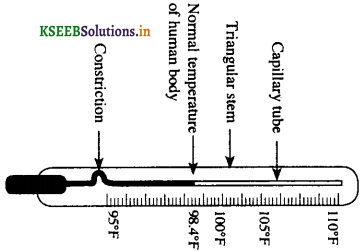A clinical thermometer is constructed in the same way as a regular laboratory mercury thermometer. The capillary tube, however, has a small constriction (twist) just above the mercury bulb. This constriction prevents the back flow of mercury when the thermometer is taken out of patient’s mouth. A clinical thermometer has a very small range, i.e., 35 °C to 42 °C.Question 31.
What are the precautions to be taken while using a clinical thermometer?
The following precautions must be taken while using a clinical thermometer:

1. The thermometer should be sterilized after each use to prevent cross contamination or possible infection. This is done by washing it and then dipping it in an antiseptic lotion.
2. The clinical thermometer should be jerked a few times before it is used to measure the temperature of the body. This is necessary to push the mercury back into the bulb.
3. Ensure that before use the mercury level is below 35 °C.
4. Clinical thermometer should not be washed with hot water.
5. The thermometer should not be held by the bulb while taking the reading.

Question 32.
The thermometer should not be washed in hot water. Give reason.
A clinical thermometer should never be washed in hot water as its temperature range is only 35 °C to 42 °C. If the thermometer is exposed to temperature above 42 °C, it is likely to burst.

Question 33.
What is the range of a clinical thermometer?
The range of a clinical thermometer is from 35 °C to 42 °C.

Question 34.
Why is the range of a clinical thermometer between 35 °C and 42 °C?
The normal temperature of the human body is about 37 °C or 98.4 °F. Our body temperature cannot fall below 35 °C or rise above 42 °C. Hence, the range of clinical thermometer is between 35 °C to 42 °C.

Question 35.
Why can’t we use a clinical thermometer to measure the temperature of hot oil or boiling water?

OR

Clinical thermometer is not suitable for measuring very high temperatures. Why?
The range of a clinical thermometer is 35 °C to 42 °C. The temperature of boiling water or hot oil is much higher than 42 °C. If we put the mercury bulb of a clinical thermometer into boiling water, the bulb breaks. Hence, a clinical thermometer is not suitable to measure the temperature of hot oil or boiling water.Question 36.
Alcohol is used as thermometric liquid to measure temperatures below 78 °C. Why?
Alcohol has a low boiling point of about 78 °C and therefore cannot be used to measure high temperatures. (Note: The boiling point of alcohol is 78 °C and not 38 °C as given in the text book.)

Question 37.
List the three commonly used temperature scales.
The commonly used temperature scales are

• Celsius scale of temperature
• Fahrenheit scale of temperature, and
• Kelvin scale of temperature.

Question 38.
Describe the construction of Celsius scale of temperature.
In Celsius scale of temperature, the freezing point of pure water is taken as 0 °C and the boiling point of pure water is taken as 100 °C. The distance between these two fixed temperatures is divided into 100 equal divisions. Each division on this scale is called one degree centigrade or one degree celsius and is written as °C.

Question 39.
Describe Fahrenheit scale of temperature.
In Fahrenheit scale of temperature, the melting point of ice is taken as 32 °F and the boiling point of pure water is taken as 212 °F. The distance between these two fixed temperatures is divided into 180 equal divisions.

Question 40.
Describe the features of Kelvin scale of temperature.
In Kelvin scale, the temperature of the melting point of ice is 273 K and the boiling point of water is 373 K. As in the Celsius scale there are 100 gradations between these two points. Hence the Kelvin scale of temperature is similar to Celsius scale of temperature.

Question 41.
Draw a neat diagram showing the comparison of the three scales of temperature.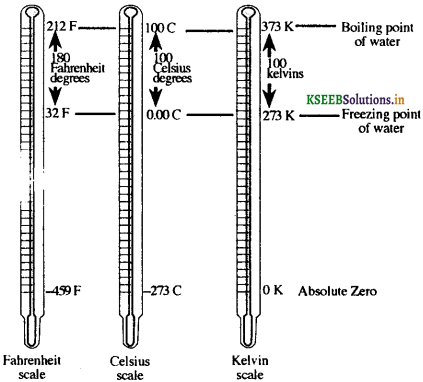Question 42.
Give the values of lowest possible temperature in the Celsius scale, Fanrenheit scale and Kelvin scale.

• Lowest possible temperature on Celsius scale: -273 °C
• Lowest possible temperature on Fahrenheit scale: -459 °F
• Lowest possible temperature on Kelvin scale: 0 K.

Question 43.
Give a formula to convert a temperature on Celsius scale to Kelvin scale and vice-versa.
The relationship between Celsius scale and Kelvin’s scale of temperatures can be expressed by one of the two formulae

• K = °C + 273
• °C = K – 273.

Question 44.
Convert 100 °C into Kelvin scale.
K = °C + 273
= 100 + 273
= 373.
100 °C corresponds to 373 K.

Question 45.
Express 77 K in Celsius scale.
C = K – 273
= 77 – 273
= -196
77 K corresponds to -1% °C.

Question 46.
Give the formula to convert Celsius scale into Fahrenheit scale.
C = f (F – 32)
Note:

• °F to °C → Deduct 32, then multiply by 5, then divide by 9.
• °C to °F → Multiply by 9, then divide by 5, then add 32.

Question 47.
What is melting point of a solid? Give two examples.
The fixed temperature at which a substance changes from solid to liquid state is called its melting point. eg. Melting point of copper is 1083 °C. The melting point of water is 0 °C.

Question 48.
The melting point of aluminium is 659 °C. What is the meaning of this statement?
This statement means that aluminium changes from solid to liquid state at the fixed temperature of 659 °C.Question 49.
Describe a simple experiment to determine the melting point of ice.
Take a bottle fitted with a one-holed rubber cork. Fit a glass funnel through the hole. Place a few ice cubes in the funnel. Suspend a thermometer such that its mercury bulb is surrounded by ice cubes.

The ice begins to melt. After about 10 minutes, the temperature in the thermometer becomes stable. Note down this temperature. This temperature is the melting point of ice.Question 50.
What is meant by boiling point of a substance? Give the boiling point of water.
The fixed temperature at which a substance in liquid state changes to vapour state is called its boiling The boiling point of water is 100 °C.

Question 51.
Describe an experiment to determine the boiling point of water.
Take some amount of water in a round bottomed flask fitted with a two-holed rubber cork. Insert a thermometer through one of the holes and a glass tube through the other. Make sure that the mercury bulb is fully immersed in water. Add a few fragments of broken porcelain.

Broken porcelain pieces help in uniform heating of water. Heat the flask until water begins to boil. When the temperature becomes stable, note down the temperature. This is the boiling point of water.Question 52.
Give the melting point and boiling point of some commonly known substances.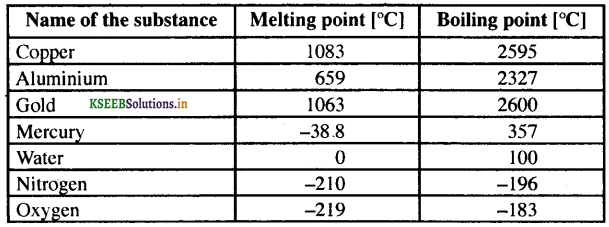Multiple Choice Questions

Question 1.
When an object is heated, the molecules that make up the object
(a) begin to move faster
(b) lose energy
(c) become heavier
(d) become lighter
(a) begin to move fasterQuestion 2.
The temperature of an object is an indicator of
(a) the total energy of the molecules of the object
(b) the average energy of the molecules of the object
(c) the total velocity of the molecules of the object
(d) the average kinetic energy of the molecules of the object
(d) the average kinetic energy of the molecules of the object

Question 3.
A and B are two objects. The temperature of A is higher than that of B. This means that
(a) the molecules of A move faster on an average than the molecules of B
(b) the total energy of A is higher than the total energy of the molecules of B
(c) the average potential energy of A is higher than that of B
(d) the heat content of A will always be higher than that of B
(a) the molecules of A move faster on an average than the molecules of B

Question 4.
One litre of water at 30 °C is mixed with one litre of water at 50 °C. The temperature of the mixture will be
(a) 80 °C
(b) more than 50 °C but less than 80 °C
(c) between 30 °C and 50 °C
(d) less than 30 °C
(c) between 30 °C and 50 °C

Question 5.
The two temperature scales with the same interval step size are the
(a) Celsius and Fahrenheit.
(b) Fahrenheit and Kelvin.
(c) Kelvin and Celsius.
(d) All three scales have same interval size
(c) Kelvin and Celsius.

Question 6.
The freezing point of water in Kelvin scale is
(a) 0 K
(b) 273 K
(c) 0 °K
(d) 273 °K.
(b) 273 K

Question 7.
The range of temperature for a clinical thermometer is
(a) 0 °C to 42 °C
(b) 42 °C to 100 °C
(c) 35 °C to 42 °C
(d) 35 °C to 100 °C
(c) 35 °C to 42 °C

Question 8.
The average kinetic energy of the molecules of a body indicates
(a) the heat contained in it
(b) its temperature
(c) its mass
(d) its physical state
(b) its temperatureQuestion 9.
The constriction in the capillary tube of a clinical thermometer is
(a) to allow mercury’ to expand uniformly.
(b) enable the measurement of temperature below 40 °C.
(c) to prevent the mercury from flowing back quickly into the bulb.
(d) prevent bursting of the mercury bulb due to high pressure.
(c) to prevent the mercury from flowing back quickly into the bulb.

Question 10.
Heat produced by rubbing two objects involves
(a) conversion of mechanical energy into heat energy
(b) conversion of heat energy into mechanical energy
(c) decrease in the kinetic energy of the molecules
(d) increase in volume of the objects rubbed together.
(a) conversion of mechanical energy into heat energy

Fill In The Blanks

(1) The degree of hotness or coldness of an object is called temperature
(2) The temperature of boiling water in Celsius scale is 100 °C
(3) The fixed temperature at which a substance changes from solid state to liquid state is called it melting point
(4) The S.I. unit of heat is called joule
(5) An instrument used to measure the temperature of a substance is called thermometer
(6) A thermometer that has a constriction in its capillary tube is clinical thermometer
(7) A substance changes from liquid to gas at constant temperature. It is called boiling point

True or False Statements

State whether the following statements are true or false. Correct the false statements.

(1) Liquids expand on heating. [True]

(2) The unit of heat and temperature are the same. [False]
Corrected statement: The S.I. unit of heat is joule and that of temperature is kelvin.

(3) Bimetallic strip is used in an electric fan. [False]
Corrected statement: Bimetallic strip is used in an electric iron/boiler.Match The Following

 A B 1. Formation of ice on water bodies in extreme cold conditions (a) Kelvin scale 2. Rise of mercury level in thermometer (b) Sudden contraction 3. Automatic electric iron (c) Celsius scale 4. Cracking of hot glass plate when a drop of cold water falls on it (d) Boiling point (e) Bimetallic strip (f) Thermal expansion (g) Anomalous expansion of water